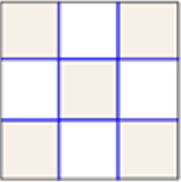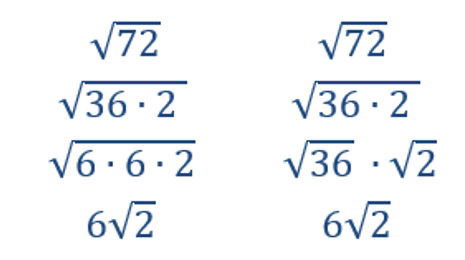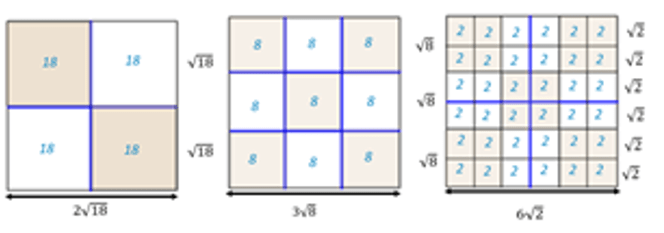## Visualizing High School Examples

An area model can be used for discussions related to squares and square roots.The area of the square is 9 and the length of each side is 3.

A ‘familiar’ area model can also be used when discussing simplifying square roots.

Symbolic or abstract manipulations to simplify or find the exact value involves no visuals. (see the example of simplifying the square root of 72 below)Consider the question that involves the following area model visual.The area of each of the three big squares is 72 u2.

What is the length of each side?

Solution:All three numbers still represent the length of the side.

A better question is why would we simplify? It is about 8.5.

• “Simplifying” is just splitting the square up into the smallest squares.